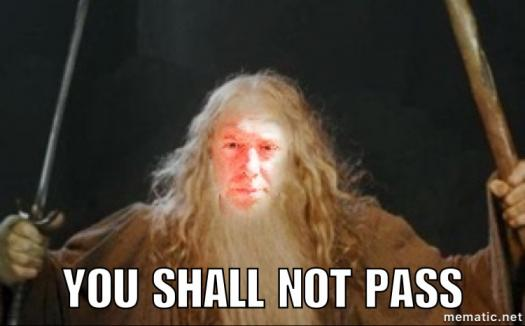# Section 6-1 To 6-3 Quiz

7 Questions | Total Attempts: 45Settings.

• 1.
Find the measure of each exterior angle of a regular 56-gon.  Round to the nearest tenth.
• A.

3.2

• B.

6.4

• C.

173.6

• D.

9720

• 2.
Given BE=2x + 6 and ED=5x - 12 in parallelogram ABCD, find BD.
• A.

6

• B.

12

• C.

18

• D.

36

• 3.
Find the measure of Angle W in parallelogram RSTW.
• A.

17

• B.

33

• C.

55

• D.

125

• 4.
Find the sum of the measures of the interior angles of a 48-gon.
• A.

172.5

• B.

360

• C.

8280

• D.

8640

• 5.
Find x.
• 6.
ABCD is a parallelogram where the measure of Angle A=138 degrees.  Find the measure of Angle B.
• 7.
Determine whether ABCD is a parallelogram if AB=6, BC=12, CD=6, and DA=12.  Answer Yes or No.Back to top